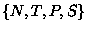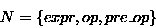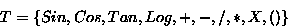Next: Genetic Algorithm for Developing Up: Grammatical Evolution : Evolving Previous: Introduction

# Backus Naur Form

Backus Naur Form (BNF) is a notation for expressing the grammar of a language in the form of production rules. BNF grammars consist of terminals, which are items that can appear in the language, i.e +, - etc. and non-terminals, which can be expanded into one or more terminals and non-terminals. A grammar can be represented by the tuple,, where N is the set of non-terminals, T the set of terminals, P a set of production rules that maps the elements of N to T, and S is a start symbol which is a member of N. For example, below is a possible BNF for a simple expression, whereS = <expr>

And P can be represented as:

(1) <expr> ::= <expr> <op> <expr>     (A)
| ( <expr> <op> <expr> ) (B)
| <pre-op> ( <expr> )    (C)
| <var>                  (D)

(2) <op> ::= + (A)
| - (B)
| / (C)
| * (D)

(3) <pre-op> ::= Sin (A)
| Cos (B)
| Tan (C)
| Log (D)

(4) <var> ::= X


Unlike a Koza-style approach, there is no distinction made at this stage between what he describes as functions (operators in this sense) and terminals (variables in this example), however, this distinction is more of an implementation detail than a design issue.

Whigham [Whigham 96] also noted the possible confusion with this terminology and used the terms GPFunctions and GPTerminals for clarity. We will also adopt this approach, and use the term terminals with its usual meaning in grammars.

Table 1 summarizes the production rules and the number of choices associated with each. When generating a sentance for a particular language, one must choose carefully which productions are to used, as, depending on the choices made, a sentance may be quite different from the desired one, possibly even of a different length.

Table 1: The number of choices available from each production rule.
 Rule no. Choices 1 4 2 4 3 4 4 1

We propose to use a genetic algorithm to control what choices are made at each juncture, thus allowing the GA to control what production rules are used. In this manner, a GA can be used to generate any manner of code in any language.Next: Genetic Algorithm for Developing Up: Grammatical Evolution : Evolving Previous: Introduction
Red Hat Linux User
1998-10-02